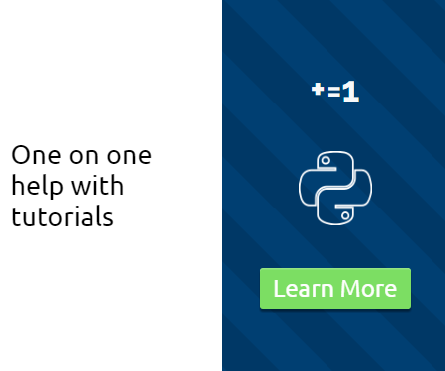SYS module Python Tutorial

This tutorial covers some of the basics to the sys module in Python 3. The sys module allows you to use stdin() and stdout(), as well as stderr(), but, most interestingly, we can utilize sys.argv(). To many, this is a confusing concept, but it is pretty simple and very useful once you learn it. The idea of sys.argv is to allow you to pass arguments through to Python from the command line.

This ability acts as a bridge to the ability to communicate between Python and other languages, which can then communicate back through the shell to interact.

With stdout and stdin, we can pass messages and errors through to the command line, or just use it for logging purposes.

Here is some basic code that matches the video:

```import sys

sys.stderr.write('This is stderr text\n')
sys.stderr.flush()
sys.stdout.write('This is stdout text\n')
```

Above, we have some of the basic stderr and stdout statements. Try them out in both a shell like bash or cmd.exe, then also try them in your Python shell.

```def main(arg):
print(arg)

main(sys.argv)
```

This code utilizes argv, which allows you to pass through arguments from the shell to your Python script or program. You will get an error if you run this without some arguments from the shell. You can always put an if-statement that asks whether sys.argv has a length greater than, for example, one, since here, we are looking for at least two.

Keep in mind that the above code is really only able to shine when you open up your command line / bash, run the script with python scriptname.py, and then add commands to it. Naturally, the script's name will be the first in the list of argv, but then anything else passed through after will be subsequent on the list. Definitely a fun tool to use, and a great way to communicate between languages and other programs.

The next tutorial:• Python Introduction

• Print Function and Strings

• Math with Python

• Variables Python Tutorial

• While Loop Python Tutorial

• For Loop Python Tutorial

• If Statement Python Tutorial

• If Else Python Tutorial

• If Elif Else Python Tutorial

• Functions Python Tutorial

• Function Parameters Python Tutorial

• Function Parameter Defaults Python Tutorial

• Global and Local Variables Python Tutorial

• Installing Modules Python Tutorial

• How to download and install Python Packages and Modules with Pip

• Common Errors Python Tutorial

• Writing to a File Python Tutorial

• Appending Files Python Tutorial

• Reading from Files Python Tutorial

• Classes Python Tutorial

• Frequently asked Questions Python Tutorial

• Getting User Input Python Tutorial

• Statistics Module Python Tutorial

• Module import Syntax Python Tutorial

• Making your own Modules Python Tutorial

• Python Lists vs Tuples

• List Manipulation Python Tutorial

• Multi-dimensional lists Python Tutorial

• Reading CSV files in Python

• Try and Except Error handling Python Tutorial

• Multi-Line printing Python Tutorial

• Python dictionaries

• Built in functions Python Tutorial

• OS Module Python Tutorial

• SYS module Python Tutorial
• Python urllib tutorial for Accessing the Internet

• Regular Expressions with re Python Tutorial

• How to Parse a Website with regex and urllib Python Tutorial

• Tkinter intro

• Tkinter buttons

• Tkinter event handling

• Tkinter images, text, and conclusion

• CX_Freeze Python Tutorial

• The Subprocess Module Python Tutorial

• Matplotlib Crash Course Python Tutorial

• Python ftplib Tutorial

• Sockets with Python Intro

• Simple Port Scanner with Sockets